## Using LightTools’ Parametric Controls for Efficient Modeling

A LightTools model includes many inputs that describe the geometry, position, and other attributes of each component. In many systems, these attributes may be related by a mathematical relationship. LightTools provides Parametric Controls to help you to manage these quantities efficiently.

The six types of Parametric Controls are described in Chapter 6 of the LightTools Core Module User’s Guide. The examples in this tip use three of these:

• Numeric parameter: a name assigned to a constant value
• Parametric expression: a name assigned to the result of a mathematical expression, which can include aliases, numeric parameters, and other parametric expression
• Pickup: a mathematical expression used to constrain the value of an input parameter of an object

To add a numeric parameter or expression, open the Parametric Controls dialog box (Edit > Parametric Controls), right click on “Parametric Expressions,” and select from the menu.You can add a LightTools input data field as a pickup by right-clicking on the parameter and picking “Add Pickup” from the context menu. The screenshot below shows the addition of the Z coordinate of planeInterface_32 as a pickup.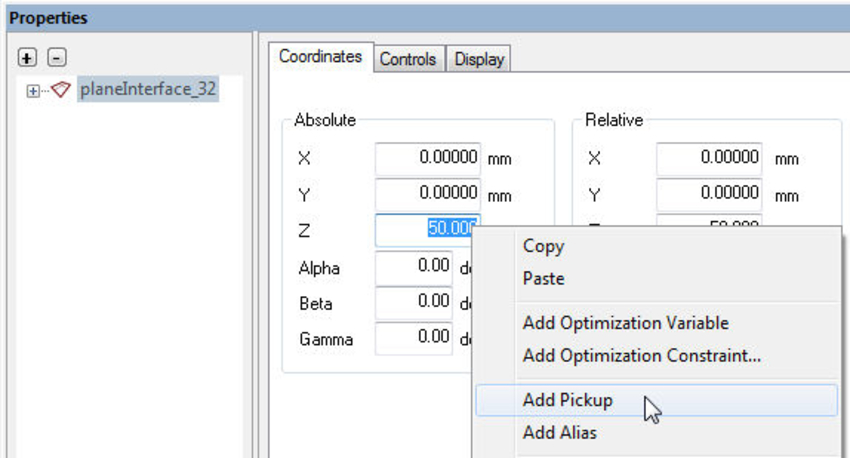## Example 1: Parabolic Mirror Parameterized by Focal Length Input

A parabolic mirror is represented in LightTools as a lens surface with a radius of curvature R and conic constant, k = -1. Defining the Focal Length as a numeric parameter, you can control the geometry of the mirror with a pickup:

Radius = 2 * Focal Length

For a mirror at the origin, you can position an indicator plane at the mirror’s focal plane by defining a second pickup:

Indicator Plane Z Location = Focal Length

As the focal length of the mirror changes, the pickups update the curvature of the mirror and keep the indicator plane at the focus.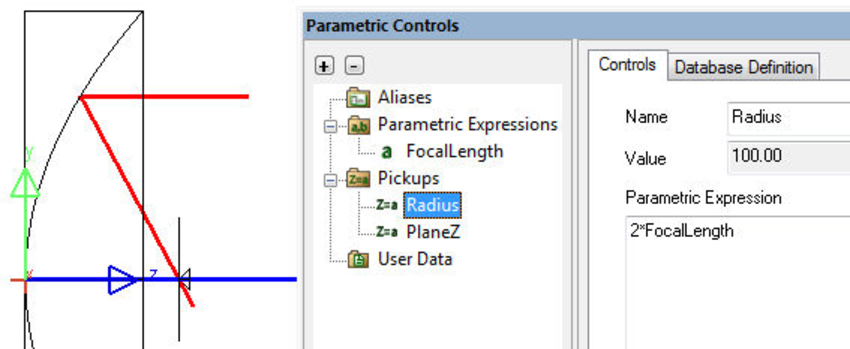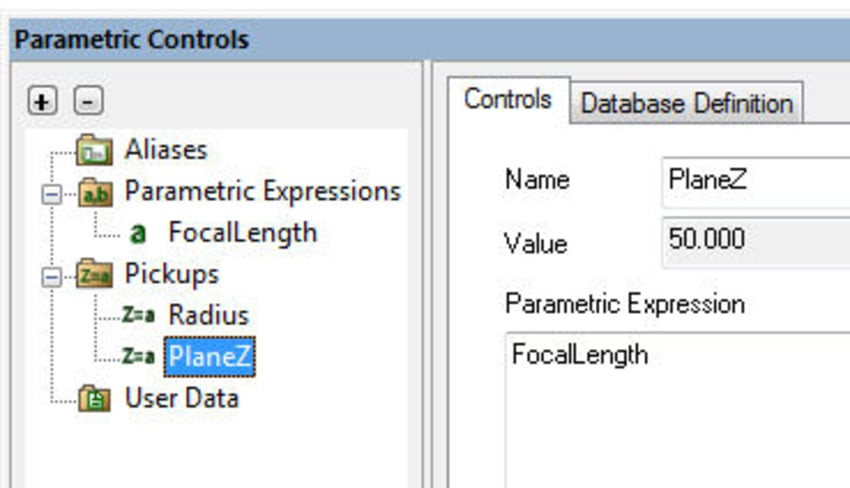## Example 2: Simplified RXI Collimating Lens

The second example consists of a plano-convex optic with index of refraction N, where the convex surface is parabolic and metalized. Light reflected from the parabolic surface may TIR at the flat side of the lens; you can adjust the thickness of the lens to position the reflected focal point at the convex surface’s vertex. The lens thickness T to maintain the focus at the vertex is F/2. This lens can be used to collimate light from a small source placed in a hole at the reflector vertex.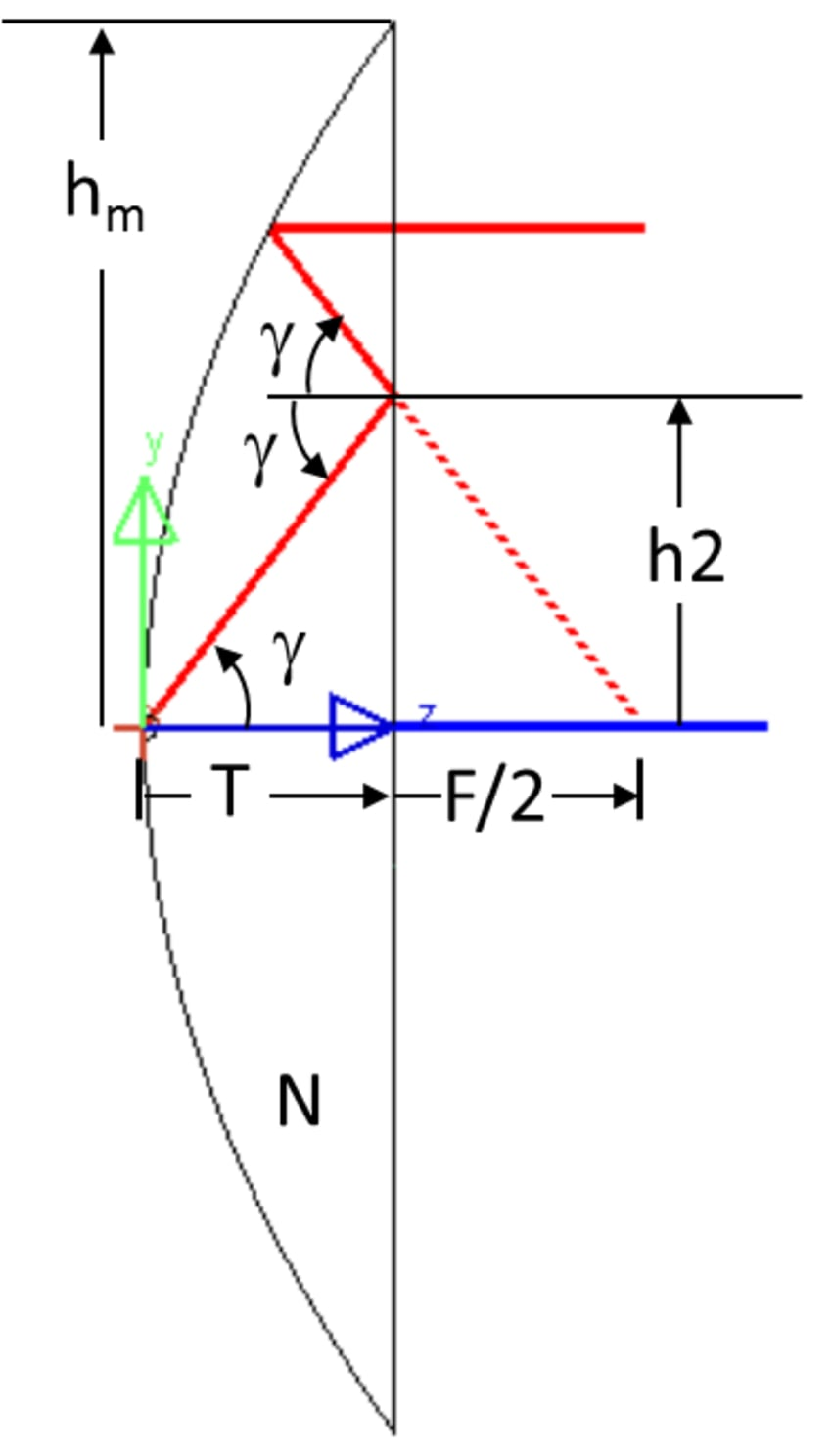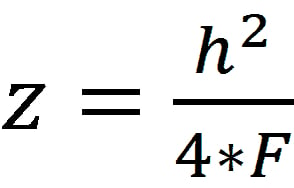The maximum height of the optic, hm, is calculated from the sag (z) equation of a parabola of revolution: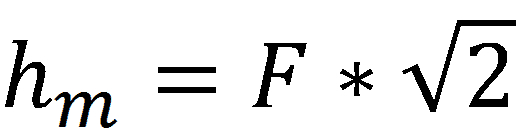Solving for hm when z = F/2 yields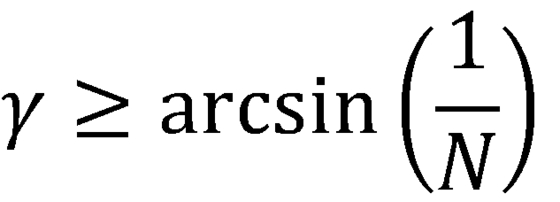The planar surface will reflect a ray by total internal reflection (TIR) if the angle of incidence of the ray, γ, satisfies the condition: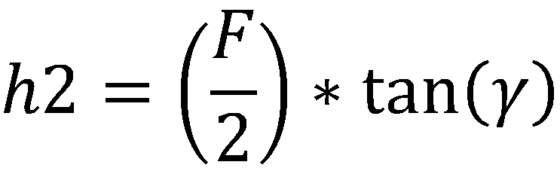Given the refractive index, N, we can compute the height, h2, of the central region where a ray will transmit inside of the region and TIR outside of the region from

Instead of transmitting the light that doesn’t TIR, we add a central property zone with a radius of h2 and a mirror optical property to the planar surface to maximize the light that reaches the focus.

To complete the example, we add a collimated NSRay fan spanning the planar surface from the edge of the mirrored region to the maximum aperture. Parametric Controls resize the ray fan to maintain this coverage.

Organizing parametric controls into groups is beneficial as the number of controls increases. After adding a group (such as an expression group), you can drag and drop a previously defined expression into that group.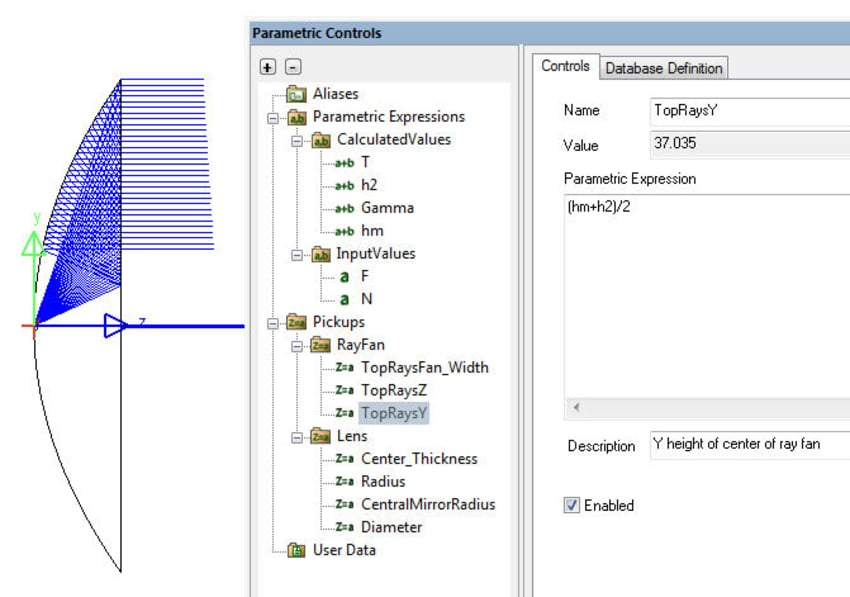Only 2 numeric parameters are input: F, the desired focal length; and N, the index of refraction of the lens material. Four Parametric Expressions calculate the geometric parameters shown in the first figure of the lens:

T = F/2,

hm = sqrt(2)*F,

Gamma = asin(1/N), and

h2 = T*tan(Gamma).

For a convex-plano lens with a parabolic LensFrontSurface at the global origin, pickups set the following parameters:

Center_Thickness = F/2,

Diameter = 2*F*sqrt(2).

Similarly, pickups are used to size the NSRay fan:

TopRaysY = (hmax + h2)/2,

TopRaysZ = T + 20, and

TopRaysFan_Width = (hmax-h2) – 0.001*hmax.

By using Parametric Controls, modifications to the full geometry can be made with the entry of just two values, allowing rapid updates of the model geometry.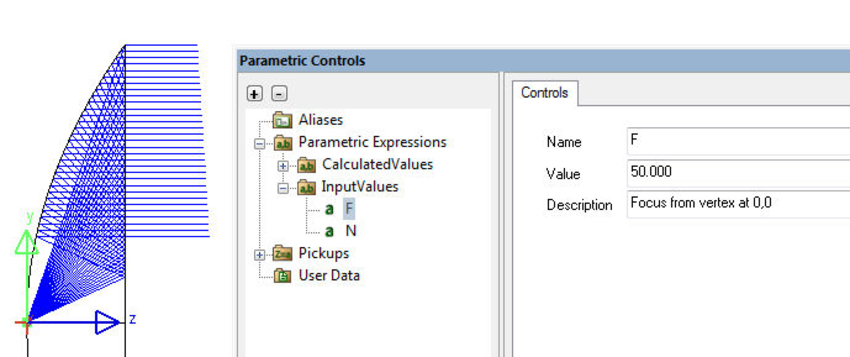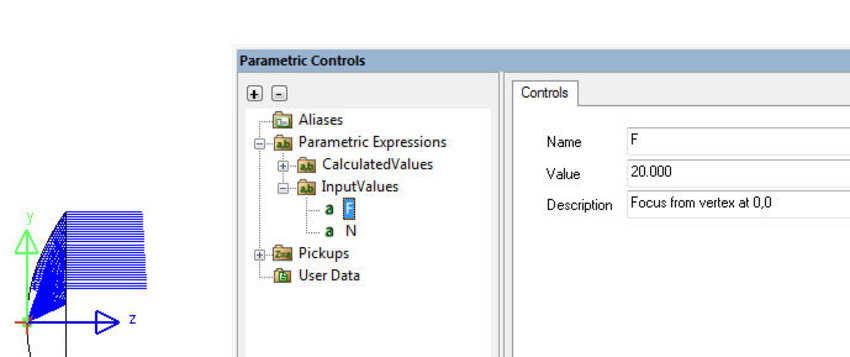The completed LightTools models for both Example 1 and Example 2 can be downloaded from the Customer Support Portal.